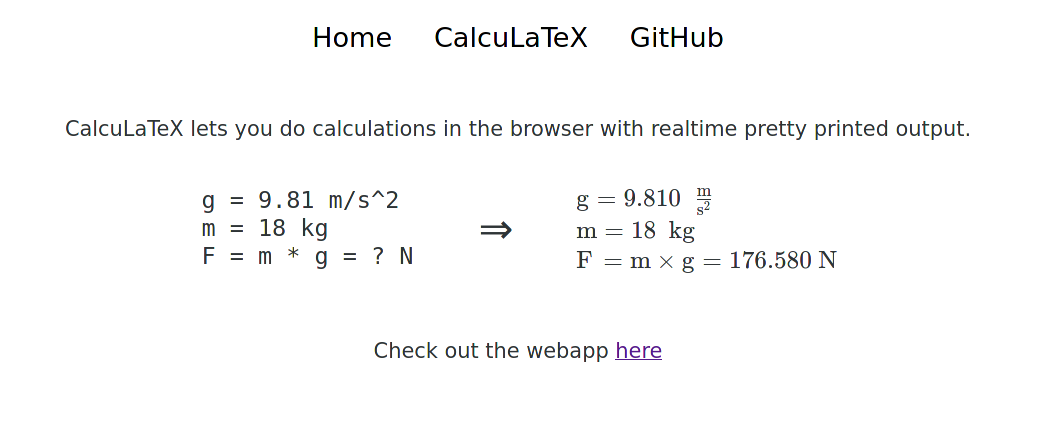# CalcuLaTeXCalcuLaTeX is a pretty printing calculator language. Its goal is to make calculations easier and more presentable with real time LaTeX rendered output, support for SI units, variables, and mathematical functions.

• Composed of an interpreter to run the calculations and a compiler to compile expressions and their answers into LaTeX
• Each expression is parsed twice, once for the interpreter, and once for the compiler, in order to make LaTeX output more closely mirror user input
• Uses a comprehensive test suite and automated CI
• CalcuLaTeX is hosted as a web app on https://calcula.tech, where it has had thousands of visitors to date.
• 300+ stars, multiple contributors on GitHub
• Written in Rust, website built with Elixir, Phoenix Liveview, and JavaScript